# How to Count Nodes in a Linked List

## How to Count Nodes in a Linked List using C Language

In this article, I am going to discuss How to Count Nodes in a Linked List using C Language with Examples. Please read our previous article, where we discussed the Recursive Function for Displaying a Linked List in C with Examples.

##### How to Count Nodes in a Linked List:

Here, we will create a function for counting the number of nodes in a linked list i.e. length of the linked list.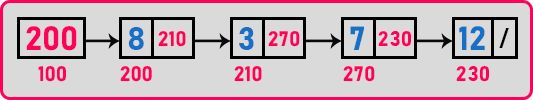We have to traverse through the linked list until we reach the last node. And every time we have to count the node. So, in this way, we can know the number of nodes in the linked lists.

int count = 0;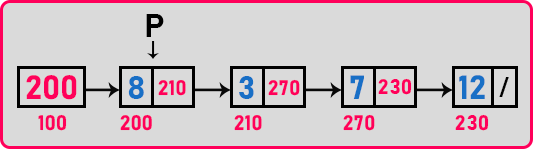We have to take one variable that stores the number of counts in a linked list, so here we have taken ‘count’ and initialized it to 0. Now p is pointing on the 1st node, so increment the count and move ‘p’ to the next node. Then ‘p’ will point on the 2nd node, so increment the count and move ‘p’ to the next node, and so on. When ‘p’ became null then stop there. This is the algorithm for counting the number of nodes. So, for this let us write a function,

```int count(struct Node *p){
int count = 0;
while(p! = 0){
count++;
p = p -> next;
}
return (count);
}
```

This is the function for calculating the length of a linked list or counting the number of nodes. Now let us analyze the time taken by this function. This function is traveling through all the nodes and simply counts. So how much time does it take for traversing all the nodes? It depends on the number of nodes. So, if n nodes are there then we will say time is O (n).

So mostly functions that are written upon linked list will be taking the order of n time. This was the iterative function to count the number of nodes. Now let us write a recursive function for counting.

##### Recursive Function 1 for Counting Nodes in a Linked List using C Language:
```int Rcount(struct Node *p){
if(p == 0)
return 0;
else
return Rcount(p->next) + 1;
}
```

The base condition for this recursive function is that ‘p’ should not be null. If ‘p’ is null then return 0 otherwise we will call the function itself with ‘p->next’ as a parameter and add 1 to this. Let us trace this recursive function:

##### Tracing Tree of Recursive Function for Counting Nodes in a Linked List: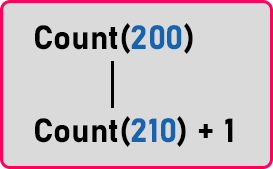First, ‘Count(200)’ will be called as ‘p’ point on the address 200. Then it will check for the condition ‘if(p == 0)’. In this case, ‘p’ is not null so it will enter in the else part. In else we will make recursive call with parameter ‘Count(p->next) + 1’. In recursive call ‘p’ is storing the address of the next node. Then we add ‘1’ to the result of the recursive call. So, the first result has to be calculated for the recursive call then only we can add ‘1’. The Addition will perform at the return time of the calls. Below is the complete tracing tree.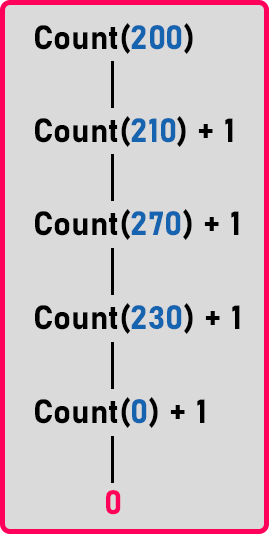So, when all the nodes will traverse then ‘p’ will point to null. When ‘p’ will point to null then we simply return ‘0’. When all the calls will be executed then the last call will return 0. Now from here, the addition will perform.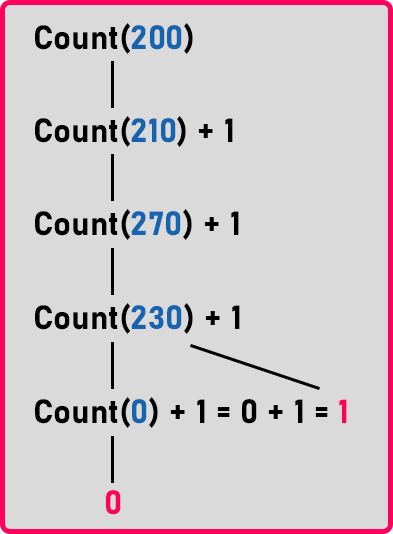As you can see in the tree, ‘Count(0)’ will return 0, then this 0 will be added to the ‘1’ of call ‘Count(230)’. So result ‘0 + 1 = 1’ will be returned to the previous call ‘Count(230)’.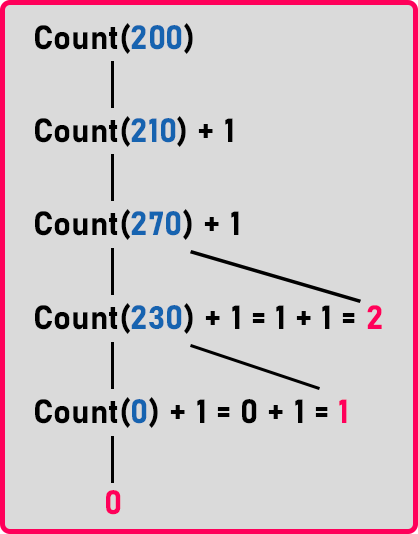Here ‘1’ is added to the result of the call ‘Count(230)’ that is ‘1 + 1 = 2’ and ‘2’ will be returned to the previous call ‘Count(270)’. In the same way, the addition will be performed in the rest of the calls. Below is the complete tracing tree: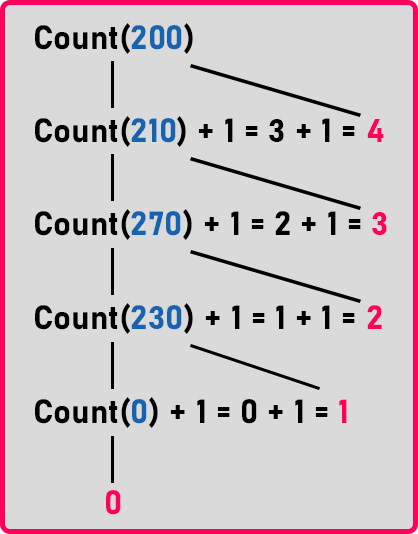So, we will get ‘4’ as the final answer. So, there are ‘4’ nodes present in the linked list. This recursive function will take,

Time Complexity: O(n)

Space Complexity: O(n)

##### Recursive Function 2 for Counting Nodes in a Linked List using C Language:
```int Rcount2(struct Node *p){
int x = 0;
if(p){
x = RCount2(p->next);
return x + 1;
}
else
return x;
}
```

This is an alternative recursive version to calculate the length of the linked list.

##### Program for Counting the Number of Nodes in a Linked List using C Language:
```#include <stdio.h>
#include <stdlib.h>
struct Node
{
int data;
struct Node *next;
}   *first = NULL;

void create(int A[], int n)
{
int i;
struct Node *t, *last;
first = (struct Node *) malloc (sizeof (struct Node));
first->data = A;
first->next = NULL;
last = first;

for (i = 1; i < n; i++)
{
t = (struct Node *) malloc (sizeof (struct Node));
t->data = A[i];
t->next = NULL;
last->next = t;
last = t;
}
}

int count(struct Node *p)
{
int c = 0;
while (p)
{
c++;
p = p->next;
}
return c;
}

int Rcount(struct Node *p)
{
if (p == 0)
return 0;
else
return count (p->next) + 1;
}

int Rcount2(struct Node *p)
{
int x = 0;
if (p)
{
x = Rcount2 (p->next);
return x + 1;
}
else
return x;
}

int main()
{
int A[] = { 8, 3, 7, 12 };
create (A, 4);
printf ("Count %d\n", count (first));
return 0;
}
```
###### Output: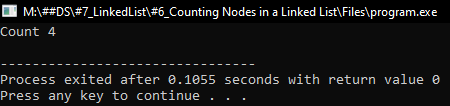In the next article, I am going to discuss how to find the Sum of all elements in a Linked list in C Language with Examples. Here, in this article, I try to explain How to Count Nodes in a Linked List using C Language with Examples and I hope you enjoy this Counting Node in a Linked List using C Language with Examples article.